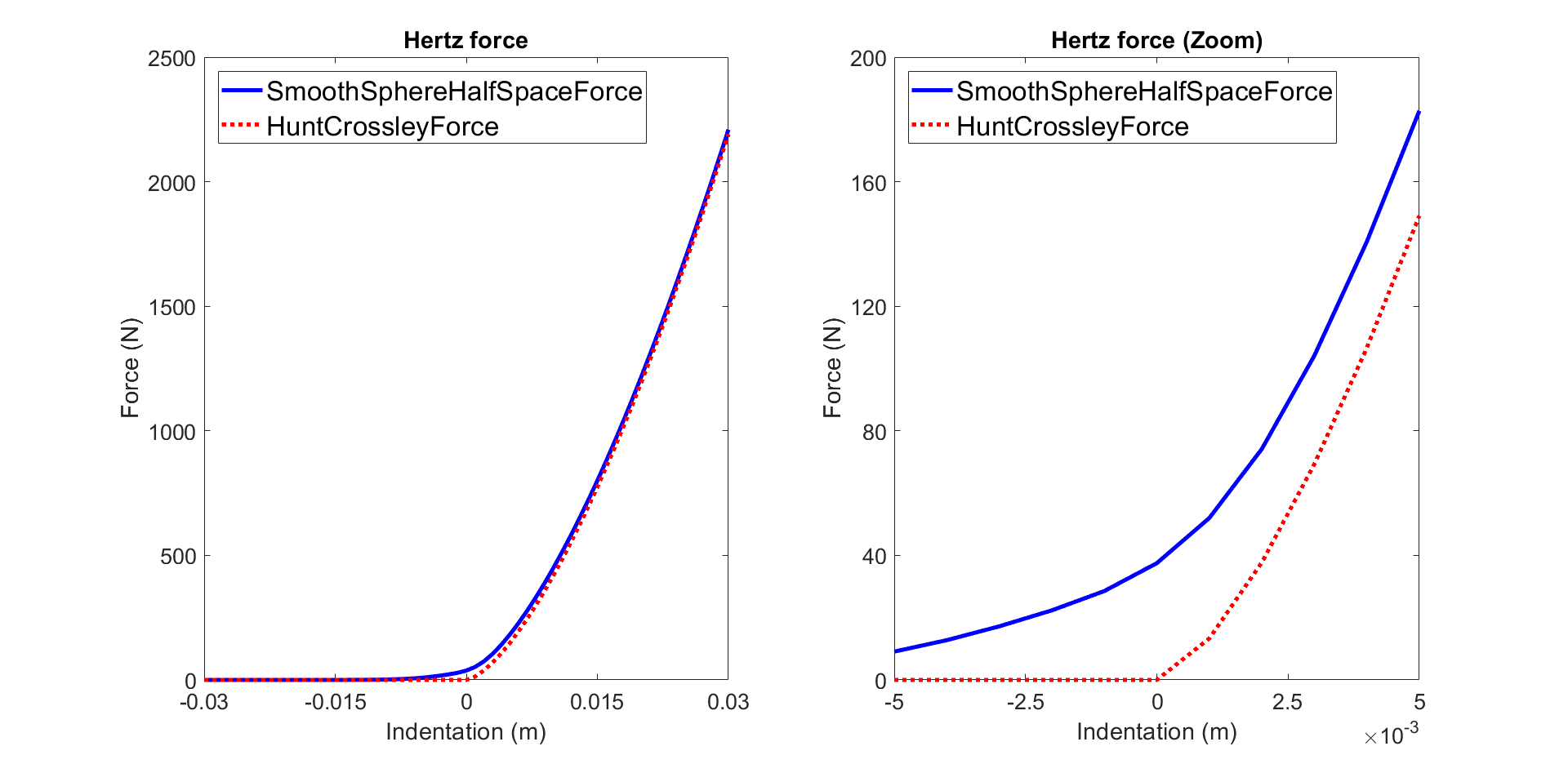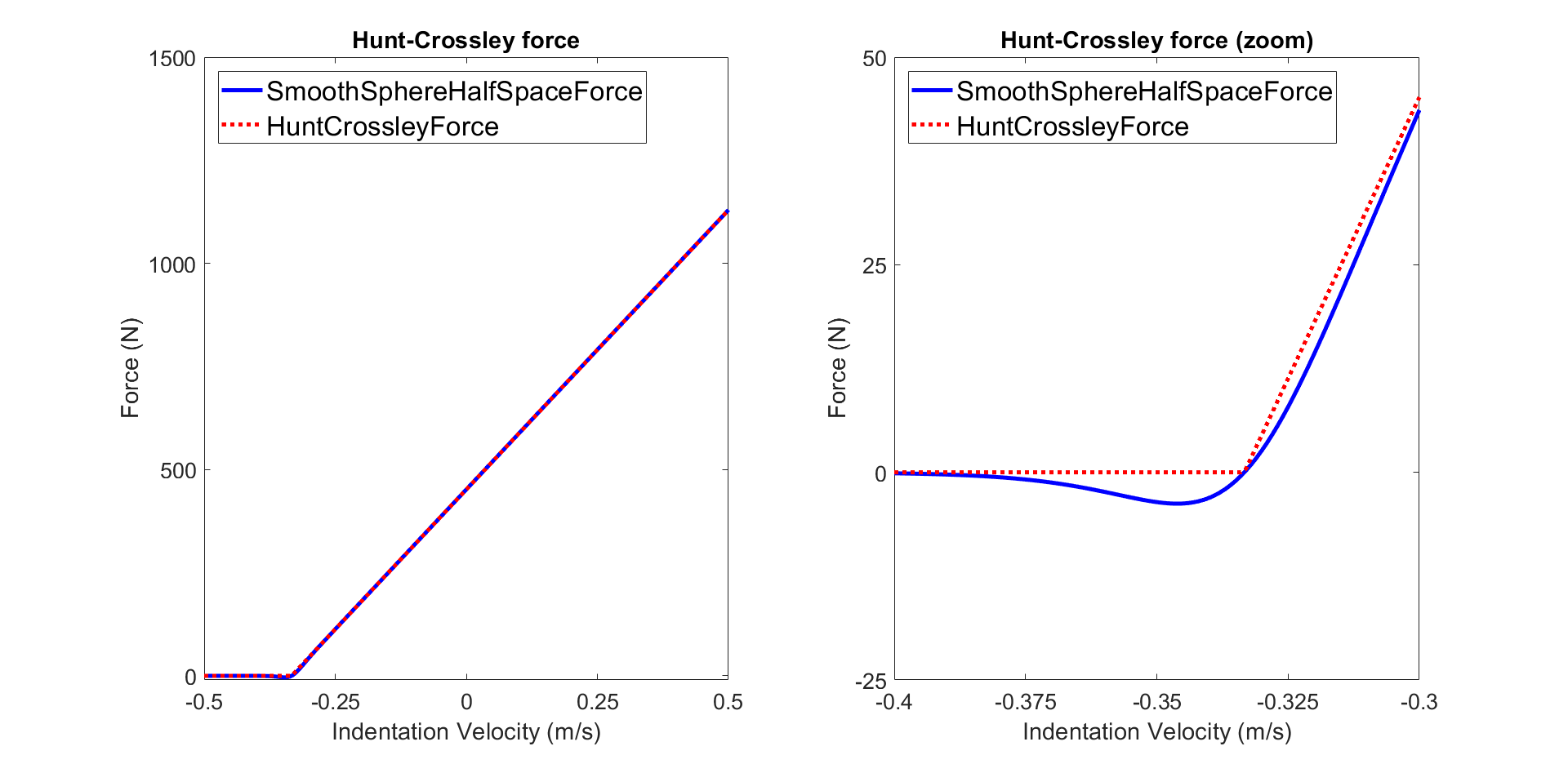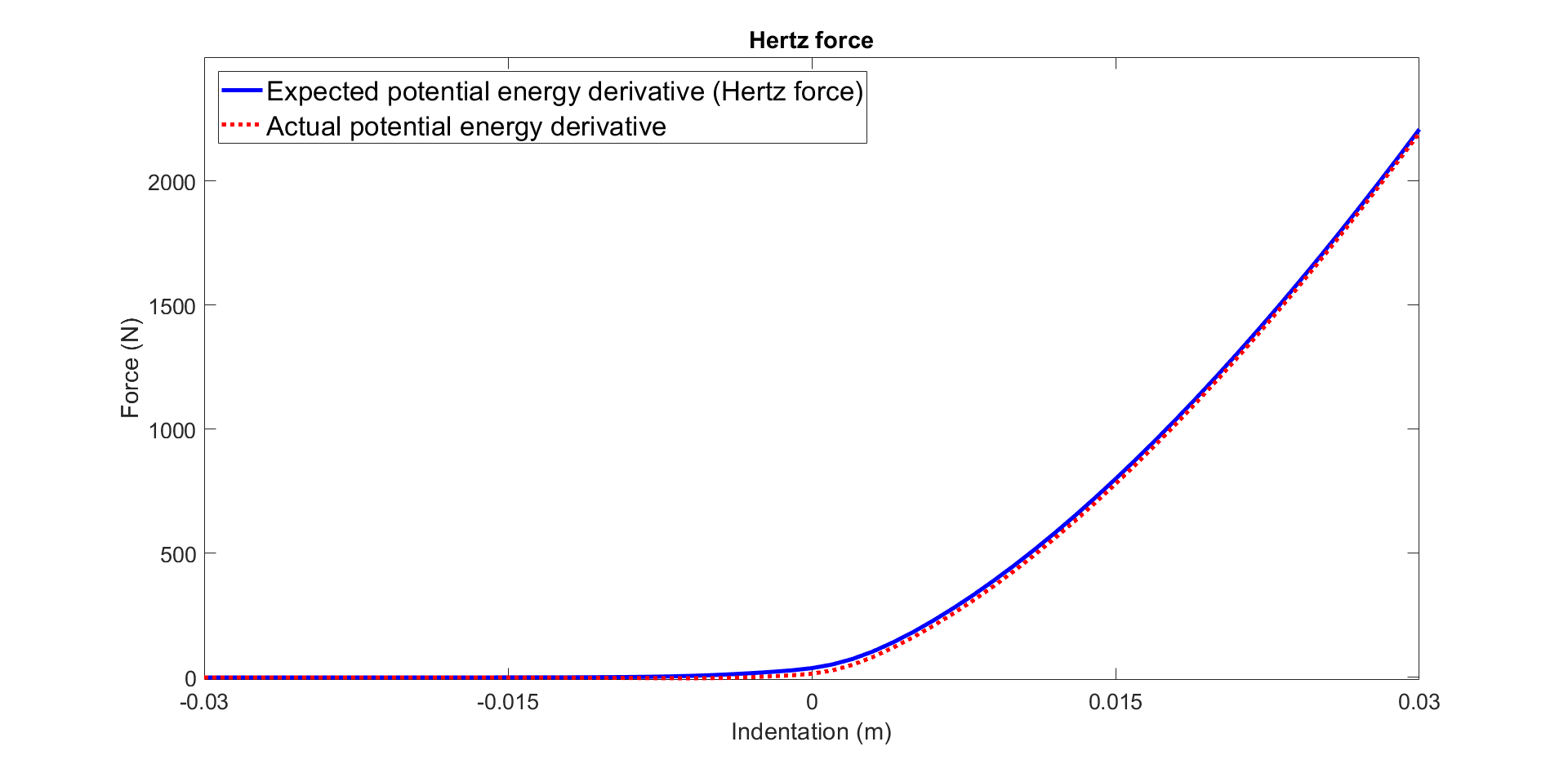Simbody  3.7
SimTK::SmoothSphereHalfSpaceForce Class Reference

This class models the forces generated by simple point contacts between a sphere and a half space. More...Inheritance diagram for SimTK::SmoothSphereHalfSpaceForce:

## Public Member Functions

SmoothSphereHalfSpaceForce (GeneralForceSubsystem &forces)
Create a smooth sphere to half space Hunt-Crossley contact model. More...

void setParameters (Real stiffness, Real dissipation, Real staticFriction, Real dynamicFriction, Real viscousFriction, Real transitionVelocity, Real cf, Real bd, Real bv)
Set the contact material parameters. More...

void setStiffness (Real stiffness)
Set the stiffness constant (i.e., plain strain modulus), default is 1 (N/m^2). More...

void setDissipation (Real dissipation)
Set the dissipation coefficient, default is 0 (s/m). More...

void setStaticFriction (Real staticFriction)
Set the coefficient of static friction, default is 0. More...

void setDynamicFriction (Real dynamicFriction)
Set the coefficient of dynamic friction, default is 0. More...

void setViscousFriction (Real viscousFriction)
Set the coefficient of viscous friction, default is 0. More...

void setTransitionVelocity (Real transitionVelocity)
Set the transition velocity, default is 0.01 (m/s). More...

void setConstantContactForce (Real cf)
Set the constant that enforces non-null derivatives, default is 1e-5. More...

void setHertzSmoothing (Real bd)
Set the parameter that determines the smoothness of the transition of the tanh used to smooth the Hertz force. More...

void setHuntCrossleySmoothing (Real bv)
Set the parameter that determines the smoothness of the transition of the tanh used to smooth the Hunt-Crossley force. More...

void setContactSphereBody (MobilizedBody bodyInput1)
Set the MobilizedBody to which the contact sphere is attached. More...

void setContactSphereLocationInBody (Vec3 locationSphere)
Set the location of the contact sphere in the body frame. More...

Set the radius of the contact sphere. More...

void setContactHalfSpaceBody (MobilizedBody bodyInput2)
Set the MobilizedBody to which the contact half space is attached. More...

void setContactHalfSpaceFrame (Transform halfSpaceFrame)
Set the transform of the contact half space in the body frame. More...

MobilizedBody getBodySphere ()
Get the MobilizedBody to which the contact sphere is attached. More...

MobilizedBody getBodyHalfSpace ()
Get the MobilizedBody to which the contact half space is attached. More...

Vec3 getContactSphereLocationInBody ()
Get the location of the contact sphere in the body frame. More...

Get the radius of the contact sphere. More...

Transform getContactHalfSpaceTransform ()
Get the transform of the contact half space. More...

SimTK_INSERT_DERIVED_HANDLE_DECLARATIONS (SmoothSphereHalfSpaceForce, SmoothSphereHalfSpaceForceImpl, Force)Public Member Functions inherited from SimTK::Force
void disable (State &) const
Disable this force element, effectively removing it from the System for computational purposes (it is still using its ForceIndex, however). More...

void enable (State &) const
Enable this force element if it was previously disabled. More...

bool isDisabled (const State &) const
Test whether this force element is currently disabled in the supplied State. More...

void setDisabledByDefault (bool shouldBeDisabled)
Normally force elements are enabled when defined and can be disabled later. More...

bool isDisabledByDefault () const
Test whether this force element is disabled by default in which case it must be explicitly enabled before it will take effect. More...

void calcForceContribution (const State &state, Vector_< SpatialVec > &bodyForces, Vector_< Vec3 > &particleForces, Vector &mobilityForces) const
Calculate the force that would be applied by this force element if the given state were realized to Dynamics stage. More...

Real calcPotentialEnergyContribution (const State &state) const
Calculate the potential energy contribution that is made by this force element at the given state. More...

Force ()
Default constructor for Force handle base class does nothing. More...

operator ForceIndex () const
Implicit conversion to ForceIndex when needed. More...

const GeneralForceSubsystemgetForceSubsystem () const
Get the GeneralForceSubsystem of which this Force is an element. More...

ForceIndex getForceIndex () const
Get the index of this force element within its parent force subsystem. More...Public Member Functions inherited from SimTK::PIMPLHandle< Force, ForceImpl, true >
bool isEmptyHandle () const
Returns true if this handle is empty, that is, does not refer to any implementation object. More...

bool isOwnerHandle () const
Returns true if this handle is the owner of the implementation object to which it refers. More...

bool isSameHandle (const Force &other) const
Determine whether the supplied handle is the same object as "this" PIMPLHandle. More...

void disown (Force &newOwner)
Give up ownership of the implementation to an empty handle. More...

PIMPLHandlereferenceAssign (const Force &source)
"Copy" assignment but with shallow (pointer) semantics. More...

PIMPLHandlecopyAssign (const Force &source)
This is real copy assignment, with ordinary C++ object ("value") semantics. More...

void clearHandle ()
Make this an empty handle, deleting the implementation object if this handle is the owner of it. More...

const ForceImpl & getImpl () const
Get a const reference to the implementation associated with this Handle. More...

ForceImpl & updImpl ()
Get a writable reference to the implementation associated with this Handle. More...

int getImplHandleCount () const
Return the number of handles the implementation believes are referencing it. More...

## Additional Inherited MembersPublic Types inherited from SimTK::PIMPLHandle< Force, ForceImpl, true >
typedef PIMPLHandle< Force, ForceImpl, PTR > HandleBase

typedef HandleBase ParentHandleProtected Member Functions inherited from SimTK::Force
Force (ForceImpl *r)
Use this in a derived Force handle class constructor to supply the concrete implementation object to be stored in the handle base. More...Protected Member Functions inherited from SimTK::PIMPLHandle< Force, ForceImpl, true >
PIMPLHandle ()
The default constructor makes this an empty handle. More...

PIMPLHandle (ForceImpl *p)
This provides consruction of a handle referencing an existing implementation object. More...

PIMPLHandle (const PIMPLHandle &source)
The copy constructor makes either a deep (value) or shallow (reference) copy of the supplied source PIMPL object, based on whether this is a "pointer semantics" (PTR=true) or "object (value) semantics" (PTR=false, default) class. More...

~PIMPLHandle ()
Note that the destructor is non-virtual. More...

PIMPLHandleoperator= (const PIMPLHandle &source)
Copy assignment makes the current handle either a deep (value) or shallow (reference) copy of the supplied source PIMPL object, based on whether this is a "pointer sematics" (PTR=true) or "object (value) semantics" (PTR=false, default) class. More...

void setImpl (ForceImpl *p)
Set the implementation for this empty handle. More...

bool hasSameImplementation (const Force &other) const
Determine whether the supplied handle is a reference to the same implementation object as is referenced by "this" PIMPLHandle. More...

## Detailed Description

This class models the forces generated by simple point contacts between a sphere and a half space.

The contact model is a smooth (i.e., twice continuously differentiable) approximation of the HuntCrossleyForce already available in Simbody. The proposed implementation was designed for use with gradient-based optimization algorithms and algorithmic/automatic differentiation. To this aim, conditional if statements were approximated by using hyperbolic tangent functions. For example, the following if statement:

`     y = 0, if x < d `
`     y = a, if x >= d `

can be approximated by:

`     f = 0.5 + 0.5 tanh(b(x-d)) `
`     y = a f `

where b is a parameter that determines the smoothness of the transition.

This force does not rely on a GeneralContactSubsystem. Instead, it assumes contact between a sphere and a half space, where the half space is defined as x > 0 (that is, all coordinates with positive x are in contact) in half space's frame. Commonly, the gravity acceleration vector is (0, -g, 0) (g > 0), and the half space represents ground. To achieve this, the half space should be rotated -90 degrees about the Z-Axis. This can be done by defining the half space frame as:

Transform exampleHalfSpaceFrame(Rotation(-0.5*Pi, ZAxis), Vec3(0))

The contact model includes components for the normal restoring force, dissipation in the material, and surface friction. The force is only applied to point contacts.

To use it, do the following:

1. Add a GeneralForceSubsystem to a MultibodySystem.
2. Add a SmoothSphereHalfSpaceForce to the GeneralForceSubsystem.
3. Add a MobilizedBody for the contact sphere and for the contact half space, and call setContactSphereBody(), setContactSphereLocationInBody(), setContactSphereRadius(), setContactHalfSpaceBody(), setContactHalfSpaceFrame(), and setParameters().

# Normal Force Components

The normal restoring force (Hertz force) is given by:

`     fh_pos = (4/3) k (R k)^(1/2) ((x^2+cf)^(1/2))^(3/2) `

where k = 0.5 E^(2/3) with E the plain strain modulus, which is assumed identical for both contacting materials (i.e., sphere and half space), x is penetration depth, R is sphere radius, and cf (default is 1e-5) is a constant that enforces non-null derivatives. To smoothly transition between periods with and without sphere-half space contact, we use a tanh function:

`     fh_smooth = fh_pos (1/2+(1/2)tanh(bd x)) `

where bd (default is 300) is a parameter that determines the smoothness of the tanh transition. The graph below compares the smooth approximation with respect to the original model.Curves produced using E=1e6, R=0.8, cf=1e-5, and bd=300

The dissipation force is combined with the normal restoring force (Hunt-Crossley force) as follows:

`     fhc_pos = fh_smooth (1+(3/2) c v) `

where c is dissipation and v is penetration rate. To smoothly transition between null and positive Hunt-Crossley force, we use a tanh function:

`     fhc_smooth = fhc_pos (1/2+(1/2) tanh(bv (v+(2/(3 c))))) `

where bv (default is 50) is a parameter that determines the smoothness of the tanh transition. The graph below compares the smooth approximation with respect to the original model.Curves produced using x=0.1, E=1e6, R=0.8, c=2, cf=1e-5, bd=300, and bv=50

# Friction Force

The friction force is given by:

` ff = fhc_smooth [min(vs/vt,1) (ud+2(us-ud)/(1+(vs/vt)^2))+uv vs] `

where vs is the slip velocity of the two bodies at the contact point (see below), vt is a transition velocity (see below), and us, ud, and uv are the coefficients of static, dynamic, and viscous friction, respectively.

The slip velocity is defined as the norm of the tangential velocity. To enforce non-null derivatives, we added the small positive constant cf (default is 1e-5):

` vs = sqrt(vtangent^2 + vtangent^2 + vtangent^2 + cf) `

where vtangent is the tangential velocity.

Because the friction force is a continuous function of the slip velocity, this model cannot represent stiction; as long as a tangential force is applied, the two bodies will move relative to each other. There will always be a nonzero drift, no matter how small the force is. The transition velocity vt acts as an upper limit on the drift velocity. By setting vt to a sufficiently small value, the drift velocity can be made arbitrarily small, at the cost of making the equations of motion very stiff.

# ContactForce

The contact force is given by:

` force = fhc_smooth*normal + ff*vtangent/vs `

where normal determines the direction of the normal to the contact half space (the normal points in the direction of contact).

# Potential energy

The potential energy is the integral of the normal restoring force (Hertz force). Due to the smooth approximation, there is no exact expression for the potential energy. We therefore made a comprise by providing an approximation for the potential energy. Specifically, we used the original expression but replaced the original Hertz force by our smooth approximation as follows:

` pe = (2/5)*fh_smooth*x `

This approximation results in the error depicted in the graph below when differentiating the potential energy with respect to the penetration, the user should thus be aware of the limitations of this model that was primarily designed for optimization problems.Curves produced using E=1e6, R=0.8, cf=1e-5, and bd=300

## ◆ SmoothSphereHalfSpaceForce()

 SimTK::SmoothSphereHalfSpaceForce::SmoothSphereHalfSpaceForce ( GeneralForceSubsystem & forces )

Create a smooth sphere to half space Hunt-Crossley contact model.

Parameters
 forces the subsystem that will own this SmoothSphereHalfSpaceForce element

## ◆ setParameters()

 void SimTK::SmoothSphereHalfSpaceForce::setParameters ( Real stiffness, Real dissipation, Real staticFriction, Real dynamicFriction, Real viscousFriction, Real transitionVelocity, Real cf, Real bd, Real bv )

Set the contact material parameters.

Parameters
 stiffness the stiffness constant (i.e., plain strain modulus), default is 1 (N/m^2) dissipation the dissipation coefficient, default is 0 (s/m) staticFriction the coefficient of static friction, default is 0 dynamicFriction the coefficient of dynamic friction, default is 0 viscousFriction the coefficient of viscous friction, default is 0 transitionVelocity the transition velocity, default is 0.01 (m/s) cf the constant that enforces non-null derivatives, default is 1e-5 bd the parameter that determines the smoothness of the transition of the tanh used to smooth the Hertz force, default is 300 bv the parameter that determines the smoothness of the transition of the tanh used to smooth the Hunt-Crossley force, default is 50

## ◆ setStiffness()

 void SimTK::SmoothSphereHalfSpaceForce::setStiffness ( Real stiffness )

Set the stiffness constant (i.e., plain strain modulus), default is 1 (N/m^2).

## ◆ setDissipation()

 void SimTK::SmoothSphereHalfSpaceForce::setDissipation ( Real dissipation )

Set the dissipation coefficient, default is 0 (s/m).

## ◆ setStaticFriction()

 void SimTK::SmoothSphereHalfSpaceForce::setStaticFriction ( Real staticFriction )

Set the coefficient of static friction, default is 0.

## ◆ setDynamicFriction()

 void SimTK::SmoothSphereHalfSpaceForce::setDynamicFriction ( Real dynamicFriction )

Set the coefficient of dynamic friction, default is 0.

## ◆ setViscousFriction()

 void SimTK::SmoothSphereHalfSpaceForce::setViscousFriction ( Real viscousFriction )

Set the coefficient of viscous friction, default is 0.

## ◆ setTransitionVelocity()

 void SimTK::SmoothSphereHalfSpaceForce::setTransitionVelocity ( Real transitionVelocity )

Set the transition velocity, default is 0.01 (m/s).

## ◆ setConstantContactForce()

 void SimTK::SmoothSphereHalfSpaceForce::setConstantContactForce ( Real cf )

Set the constant that enforces non-null derivatives, default is 1e-5.

## ◆ setHertzSmoothing()

 void SimTK::SmoothSphereHalfSpaceForce::setHertzSmoothing ( Real bd )

Set the parameter that determines the smoothness of the transition of the tanh used to smooth the Hertz force.

The larger the steeper the transition but also the more discontinuous, default is 300.

## ◆ setHuntCrossleySmoothing()

 void SimTK::SmoothSphereHalfSpaceForce::setHuntCrossleySmoothing ( Real bv )

Set the parameter that determines the smoothness of the transition of the tanh used to smooth the Hunt-Crossley force.

The larger the steeper the transition but also the more discontinuous, default is 50.

## ◆ setContactSphereBody()

 void SimTK::SmoothSphereHalfSpaceForce::setContactSphereBody ( MobilizedBody bodyInput1 )

Set the MobilizedBody to which the contact sphere is attached.

## ◆ setContactSphereLocationInBody()

 void SimTK::SmoothSphereHalfSpaceForce::setContactSphereLocationInBody ( Vec3 locationSphere )

Set the location of the contact sphere in the body frame.

Set the radius of the contact sphere.

## ◆ setContactHalfSpaceBody()

 void SimTK::SmoothSphereHalfSpaceForce::setContactHalfSpaceBody ( MobilizedBody bodyInput2 )

Set the MobilizedBody to which the contact half space is attached.

## ◆ setContactHalfSpaceFrame()

 void SimTK::SmoothSphereHalfSpaceForce::setContactHalfSpaceFrame ( Transform halfSpaceFrame )

Set the transform of the contact half space in the body frame.

## ◆ getBodySphere()

 MobilizedBody SimTK::SmoothSphereHalfSpaceForce::getBodySphere ( )

Get the MobilizedBody to which the contact sphere is attached.

## ◆ getBodyHalfSpace()

 MobilizedBody SimTK::SmoothSphereHalfSpaceForce::getBodyHalfSpace ( )

Get the MobilizedBody to which the contact half space is attached.

## ◆ getContactSphereLocationInBody()

 Vec3 SimTK::SmoothSphereHalfSpaceForce::getContactSphereLocationInBody ( )

Get the location of the contact sphere in the body frame.

 Real SimTK::SmoothSphereHalfSpaceForce::getContactSphereRadius ( )

Get the radius of the contact sphere.

## ◆ getContactHalfSpaceTransform()

 Transform SimTK::SmoothSphereHalfSpaceForce::getContactHalfSpaceTransform ( )

Get the transform of the contact half space.

## ◆ SimTK_INSERT_DERIVED_HANDLE_DECLARATIONS()

 SimTK::SmoothSphereHalfSpaceForce::SimTK_INSERT_DERIVED_HANDLE_DECLARATIONS ( SmoothSphereHalfSpaceForce , SmoothSphereHalfSpaceForceImpl , Force )

The documentation for this class was generated from the following file: